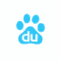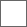### 一种计算整数平方根的简单算法

i是计数极限，那么，1/i是什么？

1/i，或者这里的1/100，也叫做网孔。意思是，如果一个数比它还小，就会从这个网中落出去。1/101

1/(100+1/100)

1/(100+1/(100+100))

100+1/(100+1/(100+1/(100+ ...

x+1/x

x+1/(x+1/(x+...

x' = x+ 1/x
x=x'

y=1+1/(1+1/(1+1/(

1.6180339887...

2+1/(2+1/(2+1/(...
= 2.41421356237309...

3+1/(3+1/(3+1/(...
=3.30277563773199...

x' = x+ k/x
x=x'

double CalculateGoldenRatio(double i, double j, int N)
{
double r = 1.0;
for (int c = 0; c < N; c++)
{
r = i + j / r;
}
return r;
}

for (int i = 1; i < 32; i++)
{
for (int j = 1; j <= i; j++)
{
double gr = CalculateGoldenRatio(i, j, 1000)
}
}i为计数的时候，没有什么东西可以对应。12楼又被莫名其妙的删除了：https://github.com/yyl-20020115/GoldenRatio#### 扫二维码下载贴吧客户端